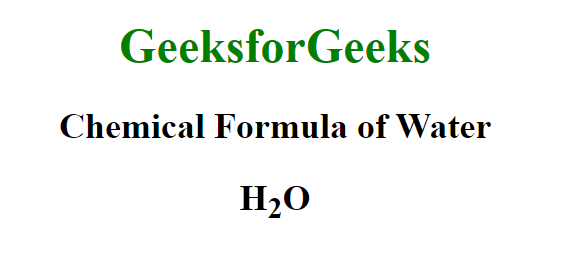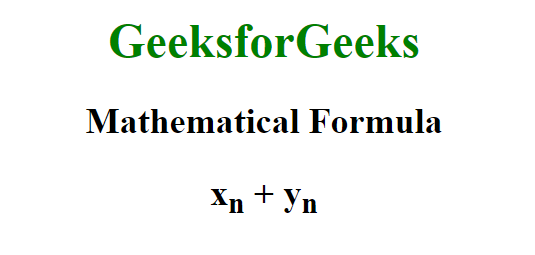Related Articles

# HTML <sub> Tag

• Last Updated : 14 Dec, 2020

This <sub> tag in HTML is used to write text below the baseline of the text in a smaller font. This tag can be used in many ways like we can denote some mathematical variables in the form of subscript text. We can also use this to denote the number of atoms of a chemical formula like the formula of glucose-c6h12o6.

Syntax:

`<sub> Contents. . . </sub>`

There are no such attributes that apply specifically to this <sub> tag. The attributes that only apply to this tag are global attributes.

Example 1:

## HTML

 ```<``html``>`` ` `<``body` `style``=``"text-align: center;"``>``     ` `    ``<``h1` `style``=``"color: green;"``>``        ``GeeksforGeeks``    ```` ` `    ``<``h2``>Chemical Formula of Water``     ` `    ``<``h2``>H<``sub``>2O```` ` ``

Output:Example 2:

## HTML

 ```<``html``>`` ` `<``body` `style``=``"text-align: center;"``>`` ` `    ``<``h1` `style``=``"color: green;"``>``        ``GeeksforGeeks``    ```` ` `    ``<``h2``>Mathematical Formula`` ` `    ``<``h2``>x<``sub``>n + y<``sub``>n```` ` ``

Output:Supported Browsers: